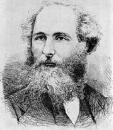Home

Lecture Notes

PHY 415: Electromagnetic Theory I
Prof. S. Teitel stte@pas.rochester.edu ---- Fall 2007

## Lecture Notes

The lecture note files correspond roughly to the material presented in a given day's lecture. But you may on occassion find the end of one day's lecture at the start of the file for the next day's lecture, so please look there if you think there might be something missing.

• Lecture 0 - A brief history of electromagnetism

• Lecture 1 - From Coulomb to Maxwell: Coulomb's law, superposition, charge density, electric field, Maxwell's equations for electrostatics

• Lecture 2 - From Coulomb to Maxwell continued: magnetostatics, Lorentz force, Biot-Savart law, local charge conservation, Faraday's law, Maxwell's correction to Ampere's law, the complete Maxwell's equations, electromagnetic waves, systems of units

• Lecture 3 - Electromagnetic scalar and vector potentials, gauge invariance, the Lorentz gauge

• Lecture 4 - Coulomb gauge, longitudinal and transverse parts of a vector field, the Greens function

Extra Notes - Review of Fourier transforms

• Lecture 5 -Electrostatics: the Coulomb problem as a boundary value problem, properties of a conductor in electrostatics, boundary conditions at a charged surface, Greens identities, uniqueness of solution

• Lecture 6 - The image charge method

• Lecture 7 - The separation of variables method: cartesian and cylindrical coordinates

• Lecture 8 - The separation of variables method: spherical coordinates

• Lecture 9 - The multipole expansion

• Lecture 10 - The magnetic dipole approximation

• Lecture 11 - The magnetic scalar potential

• Lecture 12 - Vectors and pseudovectors; the macroscopic Maxell equations: dielectrics, polarization density

• Lecture 13 - The macroscopic Maxell equations: displacement field D, bound charge, magnetic materials

• Lecture 14 - Magnetic field H, bound currents

• Lecture 15 - Boundary conditions, linear materials, Clausius - Massoti equation

• Lecture 16 - Linear dielectrics, bar magnets

• Lecture 17 - Energy conservation, Poynting vector, electrostatic and magnetostatic energy

• Lecture 18 - Momentum conservation, force on a conducting surface, capacitance matrix, inductance matrix

• Lecture 19 - Force and torque on electric and magnetic dipoles, interaction energy of electric and magnetic dipoles

• Lecture 20 - Electromagnetic waves in the vacuum, energy and momentum carried by electromagnetic waves

• Lecture 21 - Frequency dependent polarizability, frequency dependent dielectric function, electromagnetic waves in dielectrics

• Lecture 22 - Electromagnetic waves in dielectrics and conductors

• Lecture 23 - Electromagnetic waves in conductors, plasma frequency, plasma oscillations, linear, circular and elliptical polarization

• Lecture 24 - Reflection and transmission at an interface, Snell's law, total internal reflection, reflection coefficients, Brewster's angle

• Lecture 25 - Kramers-Kronig relation, Greens function for the wave equation

• Lecture 26 - Lienard-Wiechert potentials from a moving charge, radiation from an oscillating source

• Lecture 27 - Electric dipole, magnetic dipole and electric quadapole radiation, Larmor's formula

• Lecture 28 - Special relativity and electromagnetism